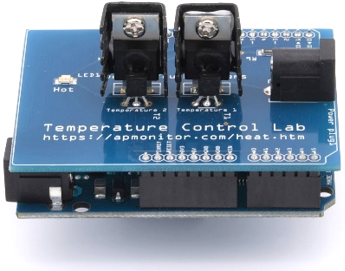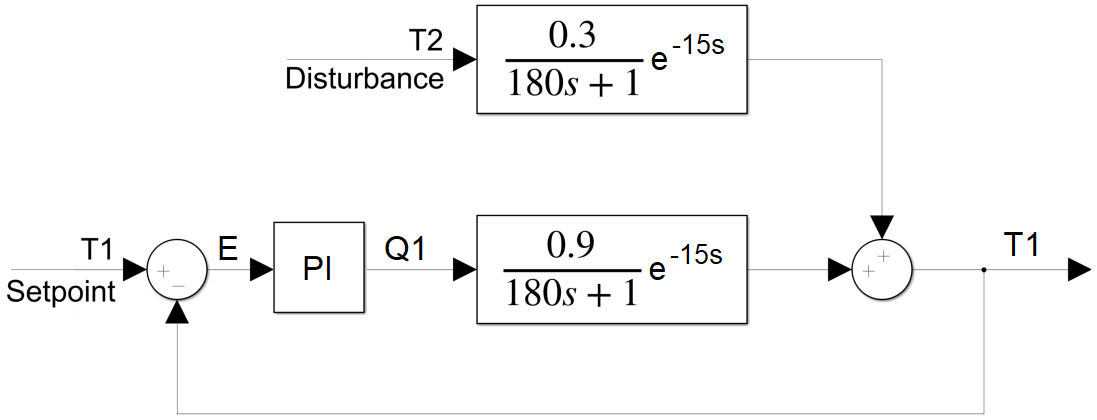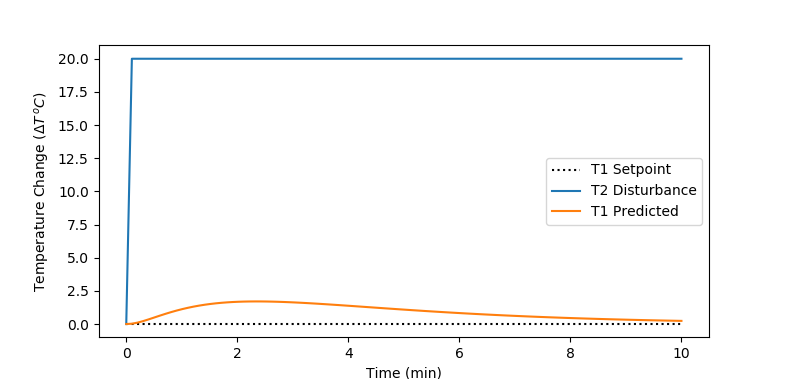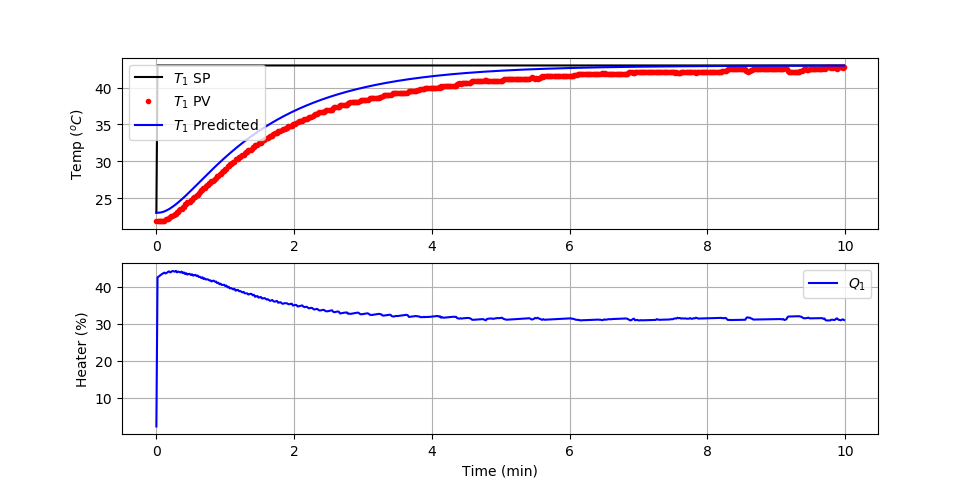## TCLab Block Diagram AnalysisObjective: Determine the closed loop response of a PI controller to a step change in setpoint. Compare the analytical solution to the closed loop response to a setpoint change.

A TCLab block diagram shows the flow of information in a feedback control system that consists of a sensor, actuator, and controller. Block diagrams are different than a process diagram in that it is a diagram of the flow of information, not necessarily how the pieces of equipment are physically placed as reviewed in the TCLab Controller Assignment. The block diagram is a collection of signals and transfer functions that transform one signal into another. Transfer functions transform an input signal into an output signal. The PI controller (K_c=2, \tau_I=180) is a transfer function G_c = K_c \frac{\tau_I s+1}{\tau_I s} that tranforms an error signal (input) into a heater value (Q1) (controller output). The process transfer function (G_p = \frac{0.9}{180s+1} e^{-15s}) receives the heater value (Q1) as an input and produces a change in temperature 1 (TA1) process variable output. The disturbance transfer function (G_d=\frac{0.3}{180s+1} e^{-15s}) has an input of temperature 2 (T2) and produces a predicted change in temperature 1 (TB1) process variable output. The two temperature outputs are added together as T1 = TA1 + TB1. The loop is created with the sensor providing information to the controller as an error (E=TSP-T1) between the setpoint and measured value. The P-only controller changes the controller output Q1 to change the temperature T1 and the cycle starts again.Block Diagram Algebra

The overall transfer function between the disturbance T2 and T1 is derived by algebraically combining the following expressions to eliminate all other signals besides the input and output of interest.

$$E(s) = T_{SP}(s)-T_1(s)$$

$$Q_1(s) = G_c(s) \, E(s) = G_c(s) \, (T_{SP}(s)-T_1(s))$$

$$T_{A1}(s) = G_p(s) \, Q_1(s) = G_c(s) \, G_p(s) \, (T_{SP}(s)-T_1(s))$$

$$T_{B1}(s) = G_d(s) \, T_2(s)$$

$$T_1(s) = T_{A1}(s)+T_{B1}(s) = G_c(s) \, G_p(s) \, (T_{SP}(s)-T_1(s)) + G_d \, T_2(s)$$

In this case, TSP(s)=0 because it is assumed that there is no change in the setpoint as the disturbance transfer function is derived.

$$T_1(s) = - G_c(s) \, G_p(s) \, T_1(s) + G_d(s) \, T_2(s)$$

The transfer function is further simplified by collecting all T1(s) terms to one side and T2(s) terms to the other.

$$T_1(s) + G_c(s) \, G_p(s) , T_1(s) = G_d \, T_2(s)$$

This simplifies to the overall closed loop transfer function for disturbance changes.

$$\frac{T_1(s)}{T_2(s)} = \frac{G_d(s)}{1+G_c(s)\,G_p(s)} = \frac{\frac{0.3}{180s+1} e^{-15s}}{1+K_c \frac{\tau_I s+1}{\tau_I s} \frac{0.9}{180s+1} e^{-15s}}$$

Time-Delay to Polynomial Form

An inverse Laplace transform is not possible with this transfer function because of the exponential terms. To compute an analytical solution to a step input, a Taylor series approximation or Padé approximation of exp(-15s) with the first 2 or 3 terms are typically used to return to polynomial form.

$$\exp(x) \approx \frac{1+\frac{1}{2}x+\frac{1}{9}x^2}{1-\frac{1}{2}x+\frac{1}{9}x^2}$$

Two terms are selected to approximate the dead-time. Additional terms improve the accuracy of the approximation but also increase the order of the polynomial.

$$\exp(-15s) \approx \frac{1-7.5 s}{1+7.5 s}$$

Taylor Series Approximation for exp

$$\exp(x) \approx \sum_{n=0}^{\infty}\frac{x^n}{n!} = 1+x+\frac{x^2}{2!}+\frac{x^3}{3!}+\dots$$

The exponential is first place in the denominator and two terms are selected to approximate the dead-time.

$$e^{-15s} = \frac{1}{e^{15s}} \approx \frac{1}{15s+1}$$

The Taylor series approximation is used for this example. The polynomial form of the transfer function is used for the analytical solution. The PI controller rejects the disturbance

Analytic Solution to PI Controller Response to +20oC T2 Disturbance$$T(t) = \left(1.14 e^{0.0178t} - 7.97 e^{0.0600t} + 6.83 e^{0.067t}\right) e^{-0.072t} S(t-1)$$

import sympy as sym
from sympy.abc import s,t,x,y,z
import numpy as np
from sympy.integrals import inverse_laplace_transform
import matplotlib.pyplot as plt

# Define inputs
T2 = 20/s*sym.exp(-s)

# Transfer functions
Kc = 2.0
tauI = 180.0
Gc = Kc * (tauI*s+1)/(tauI*s)
delay = 1/(15*s+1) # Taylor series approx
Gd = delay * 0.3/(180*s+1)
Gp = delay * 0.9/(180*s+1)

# Closed loop response
Gc = Gd/(1+Gc*Gp)

# Calculate response
T1 = Gc * T2

# Inverse Laplace Transform
t2 = inverse_laplace_transform(T2,s,t)
t1 = inverse_laplace_transform(T1,s,t)
print('Temperature Solution')
print(t1)

# generate data for plot
tm = np.linspace(0,600,100)
T2plot = np.zeros(len(tm))
T1plot = np.zeros(len(tm))

# substitute numeric values
for i in range(len(tm)):
T2plot[i] = t2.subs(t,tm[i])
T1plot[i] = t1.subs(t,tm[i])

# plot results
plt.figure()
plt.plot([0,10],[0,0],'k:',label='T1 Setpoint')
plt.plot(tm/60,T2plot,label='T2 Disturbance')
plt.plot(tm/60,T1plot,label='T1 Predicted')
plt.legend()
plt.xlabel('Time (min)')
plt.ylabel(r'Temperature Change $(\Delta T \, ^oC)$')
plt.show()

Compute T1 Analytic Solution to +20oC Setpoint Change

Using the above as an example, calculate the closed loop transfer function for a PI controller between the other input (setpoint TSP) and the output (T1). Compute the analytical solution for T1 and show predicted and measured values for a PI controller setpoint change from 23oC to 43oC. Use the PI Test Script to show the analytical and measured values for the closed loop control, modifying the line for the predicted temperature.

T1Pred = # fill in analytic solution

import numpy as np
import matplotlib.pyplot as plt
import tclab
import time

n = 600  # Number of second time points (10 min)
tm = np.linspace(0,n-1,n) # Time values
T1Pred = # fill in analytic solution

# Kc,tauI,tauD
Kc = 2.0
tauI = 180.0
tauD = 0

# PID Controller Function
def pid(sp,pv,pv_last,ierr,dt):
# Parameters in terms of PID coefficients
KP = Kc
KI = Kc/tauI
KD = Kc*tauD
# ubias for controller (initial heater)
op0 = 0
# upper and lower bounds on heater level
ophi = 100
oplo = 0
# calculate the error
error = sp-pv
# calculate the integral error
ierr = ierr + KI * error * dt
# calculate the measurement derivative
dpv = (pv - pv_last) / dt
# calculate the PID output
P = KP * error
I = ierr
D = -KD * dpv
op = op0 + P + I + D
# implement anti-reset windup
if op < oplo or op > ophi:
I = I - KI * error * dt
# clip output
op = max(oplo,min(ophi,op))
# return the controller output and PID terms
return [op,P,I,D]

lab = tclab.TCLab()
T1 = np.zeros(n)
Q1 = np.zeros(n)
# step setpoint from 23.0 to 43.0 degC
SP1 = np.ones(n)*23.0
SP1[1:] = 43.0
Q1_bias = 0.0
ierr = 0.0
for i in range(n):
T1[i] = lab.T1
[Q1[i],P,ierr,D] = pid(SP1[i],T1[i],T1[max(0,i-1)],ierr,1.0)
lab.Q1(Q1[i])
if i%20==0:
print(' Heater,   Temp,  Setpoint')
print(f'{Q1[i]:7.2f},{T1[i]:7.2f},{SP1[i]:7.2f}')
time.sleep(1)
lab.close()

# Save data file
data = np.vstack((tm,Q1,T1,SP1)).T
np.savetxt('PI_control.csv',data,delimiter=',',\

# Create Figure
plt.figure(figsize=(10,7))
ax = plt.subplot(2,1,1)
ax.grid()
plt.plot(tm/60.0,SP1,'k-',label=r'$T_1$ SP')
plt.plot(tm/60.0,T1,'r.',label=r'$T_1$ PV')
plt.plot(tm/60.0,T1Pred,'b-',label=r'$T_1$ Predicted')
plt.ylabel(r'Temp ($^oC$)')
plt.legend(loc=2)
ax = plt.subplot(2,1,2)
ax.grid()
plt.plot(tm/60.0,Q1,'b-',label=r'$Q_1$')
plt.ylabel(r'Heater (%)')
plt.xlabel('Time (min)')
plt.legend(loc=1)
plt.savefig('PI_Control.png')
plt.show()

Solution

The overall transfer function between the disturbance TSP and T1 is derived by algebraically combining the same expressions to eliminate all other signals besides the input and output of interest.

$$E(s) = T_{SP}(s)-T_1(s)$$

$$Q_1(s) = G_c(s) \, E(s) = G_c(s) \, (T_{SP}(s)-T_1(s))$$

$$T_{A1}(s) = G_p(s) \, Q_1(s) = G_c(s) \, G_p(s) \, (T_{SP}(s)-T_1(s))$$

$$T_{B1}(s) = G_d(s) \, T_2(s)$$

$$T_1(s) = T_{A1}(s)+T_{B1}(s) = G_c(s) \, G_p(s) \, (T_{SP}(s)-T_1(s)) + G_d \, T_2(s)$$

In this case, T2(s)=0 because it is assumed that there is no change in the disturbance as the setpoint transfer function is derived.

$$T_1(s) = G_c(s) \, G_p(s) \, (T_{SP}(s)-T_1(s))$$

The transfer function is further simplified by collecting all T1(s) terms to one side and TSP(s) terms to the other.

$$T_1(s) + G_c \, G_p(s) \, T_1(s) = G_c \, G_p(s) \, T_{SP}(s)$$

The closed loop transfer function is the ratio of the output to input.

$$\frac{T_1(s)}{T_{SP}(s)} = \frac{G_c \, G_p(s)}{1+G_c \, G_p(s)}$$

Analytic Solution to +20oC Setpoint Change$$T(t) = \left( 20.0 + 6.14 e^{-0.054\,t} - 26.13 e^{-0.012\,t} \right) S(t-1)$$

import sympy as sym
from sympy.abc import s,t,x,y,z
import numpy as np
from sympy.integrals import inverse_laplace_transform
import matplotlib.pyplot as plt

# Define inputs
# Setpoint step (up) starts at 1 sec
TSP = 20/s*sym.exp(-s)

# Transfer functions
Kc = 2.0
tauI = 180.0
Gc = Kc * (tauI*s+1)/(tauI*s)
delay = 1/(15*s+1) # Taylor series approx
Gp = delay * 0.9/(180*s+1)

# Closed loop response
Gc = Gc*Gp/(1+Gc*Gp)

# Calculate response
T1 = Gc * TSP

# Inverse Laplace Transform
tsp = inverse_laplace_transform(TSP,s,t)
t1 = inverse_laplace_transform(T1,s,t)
print('Temperature Solution')
print(t1)

# generate data for plot
tm = np.linspace(0,600,100)
TSPplot = np.zeros(len(tm))
T1plot = np.zeros(len(tm))

# substitute numeric values
for i in range(len(tm)):
TSPplot[i] = tsp.subs(t,tm[i]) + 23.0
T1plot[i] = t1.subs(t,tm[i]) + 23.0

# plot results
plt.figure()
plt.plot(tm/60,TSPplot,'k:',label='T1 Setpoint')
plt.plot(tm/60,T1plot,'b-',label='T1 Predicted')
plt.legend()
plt.xlabel('Time (min)')
plt.ylabel(r'Temperature Change $(\Delta T \, ^oC)$')
plt.show()

The PI Control test script is modified with the analytic solution.

T1Pred = 20 + 6.14*np.exp(-0.054*tm) - 26.13*np.exp(-0.012*tm) + 23.0

The plot shows a comparison of the predicted and measured temperature values. The ambient temperature is less than the 23oC simulated value and can be adjusted to achieve a better match to the analytic solution. However, despite the offset, the measured dynamic response of the closed-loop response is similar to the analytic solution.